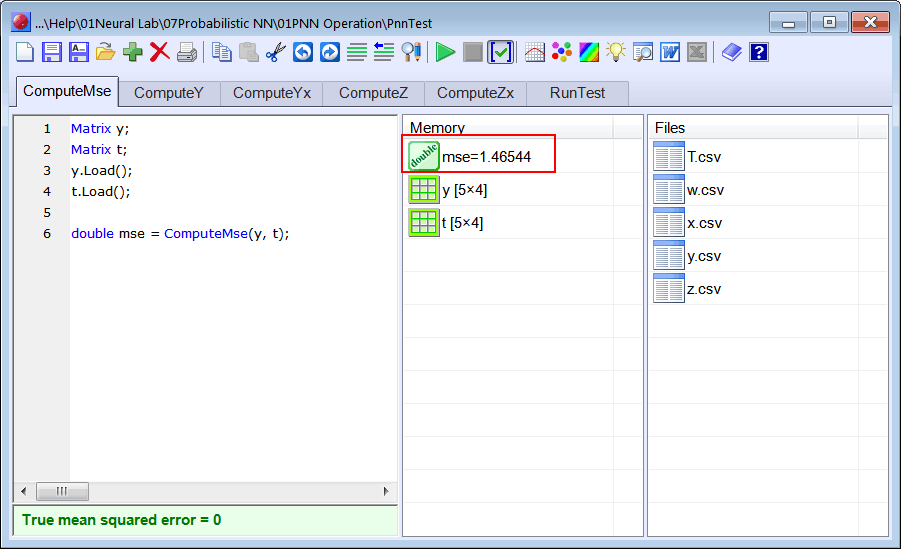# Probabilistic Neural Network Operation

## PNN Operation

The figure below shows the internal structure of a PNN. There are two main internal parts in the structure of a PNN. The first part is represented by a green box; this block computes the values of z1, z2, ...., zN using the input values (xm1, xm2, ...., x), the network weights (w1, w2, ...., wλ), and the training set input X. Thus, it can be said that each training case has an associated value of z. The second part of a PNN, displayed by a pink box, computes the output of the network.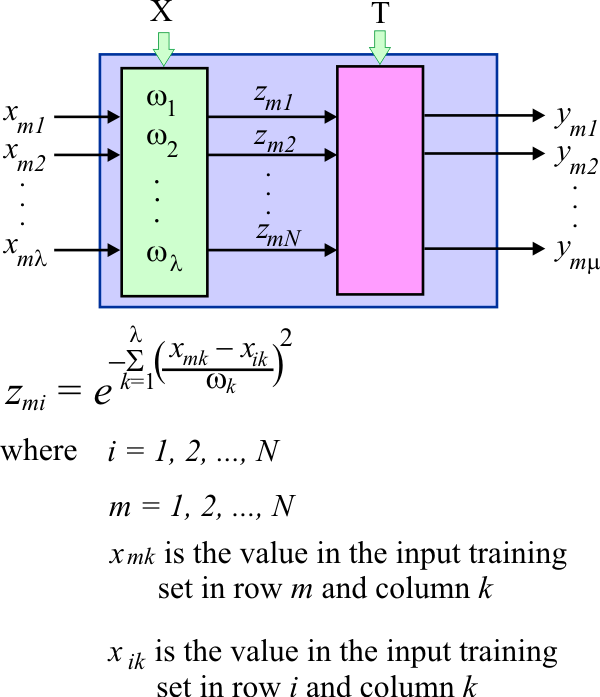## Output Calculation

The equation below shows how the output of a PNN is computed. A quick inspection to the equation reveals that the output of a PNN is a linear combination of the cases in training set target. The value of z will amplify or attenuate the training set target. Specifically, if an input value is similar to a case in the training set input, the value for of z for this training case will be close to one, and the output of the PNN will be similar to the target of this training case. In the same way, if an input is not similar to a case in the training set input, the value for of z for this training case will be close to zero, and the output of the PNN will not be influenced by the target of this training case.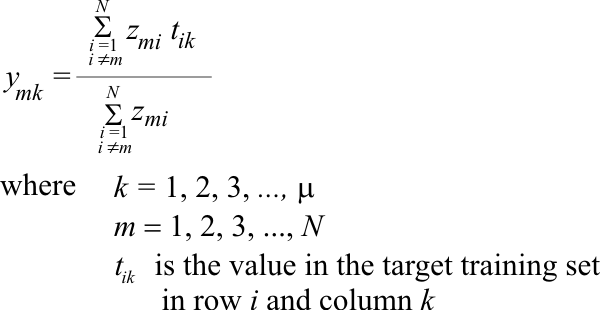Problem 1 Create a Neural Project called PnnTest to perform several test to understand the operation of Probabilistic Neural Networks, use the Main file only option. Add the The ComputZ.lab file to compute the value of z. An PNN has three inputs and four outputs as shown. Suppose that the PNN weights are as shown in the figure. For each input in the training set input compute z1, z2, z3, z4, z5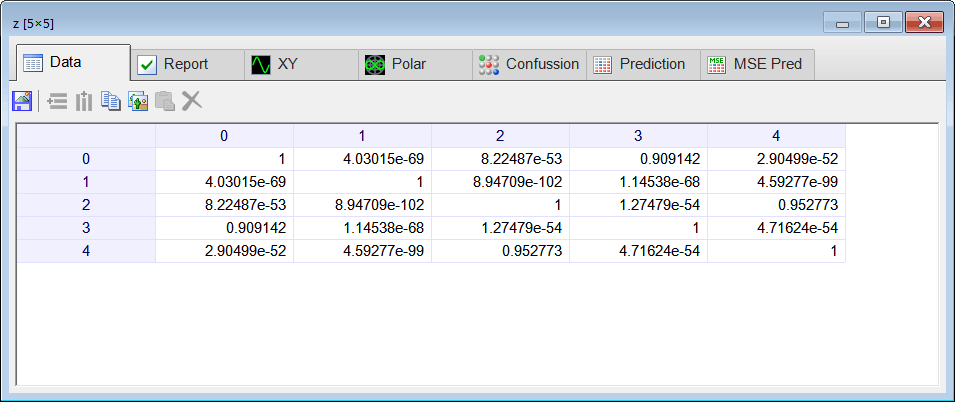Problem 2 In row zero of the z matrix there are is a one in column zero, and value of 0.909141 in column 3 which is close to one. In the same way, in row 2 there is a one in column 2, and value of 0.952773 in column 4 which is close to one. Explain the meaning of these values close to one: (a) in row 0, (b) in row 2.

 Problem 3 In row zero of the z matrix there are very small values in columns: 2, 3, and 4. Explain the meaning of these small values.

 Problem 4 Compute the output for each input in the training set input for PNN described previously.

 PnnTest\ComputeY.lab Matrix z; Matrix t; z.Load(); t.Load(); int numCases = t.GetRowCount(); ... y.Save();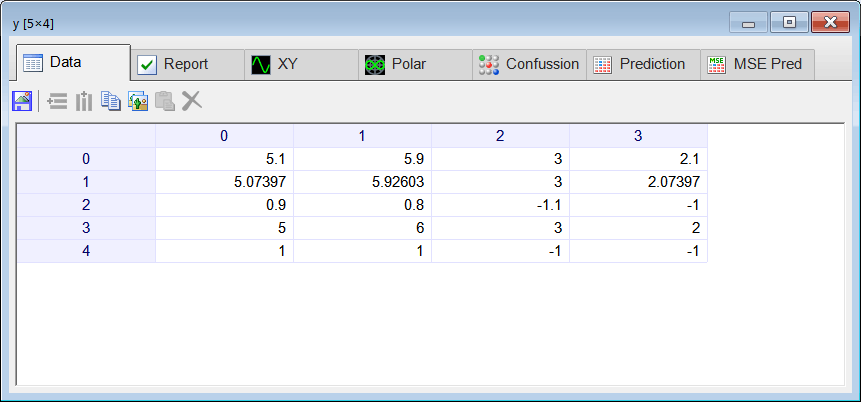Problem 5 Compute the mse for the training set by writing the ComputeMse.lab file.